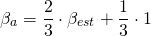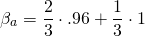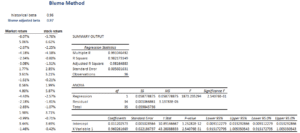Blume Method

The Blume method or Blume adjustment is a method to adjust the estimated market beta. The approach was proposed in 1975 by Marshall E. Blume in a paper on equity betas’ regression tendencies (although an earlier article published in 1971 by Blume also discusses the method). The Blume adjustment corrects the estimated market beta of a security for the tendency of market betas to revert back to 1. Thus, the method adjusts the market beta such that it is more robust and closer to the expected beta in the future. The method is similar to the Vasicek adjusted beta.

On this page, we discuss the Blume adjusted beta formula and illustrate the application using a simple example. A spreadsheet implementing the approach is also available at the bottom of the page. In the spreadsheet, we first estimate the historical beta and then adjust it.

Blume’s formula

The Blume adjustment formula is the followingThe Blume method is mainly used to adjust the beta when forecasting. In that case, since we know the market beta has a tendency to revert back to one, our estimate will be more accurate by incorporating this knowledge. The Blume adjusted beta is often referred to by analysts as the forecasted beta. The estimated beta is referred to as the historical beta.

Blume method example

Let’s turn to a simple example to illustrate the approach. Suppose we estimate market beta of a security using three years of monthly data. With 36 observations, we obtain an estimated beta of 0.96. Now, we apply Blume’s formulawhich yields a slightly higher beta of 0.97. The magnitude of the adjustment depends on the initial historical beta. Suppose we estimated a beta of 1.4. Then the adjusted beta would have been 1.26, which is a larger adjustment than in the first example. The following table implements an example in Excel. The spreadsheet can be downloaded below.Summary

We discussed the Blume adjusted beta. The adjusted beta is more robust and should be used when performing forecasts with stock market betas.